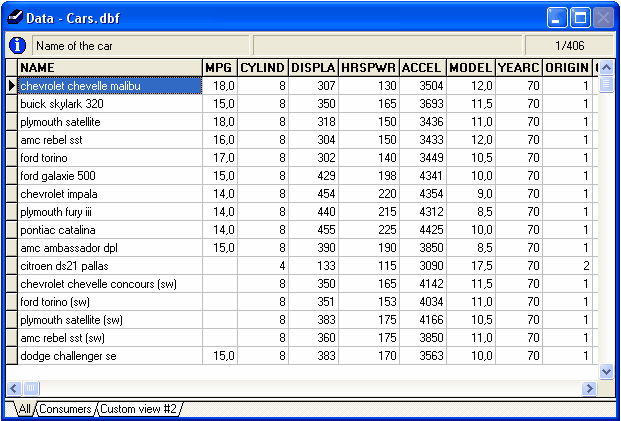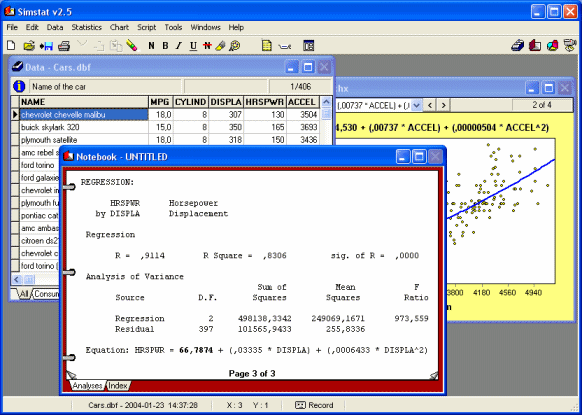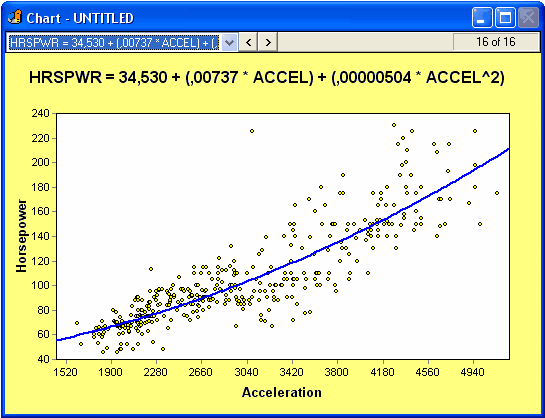## Quick purchase

Please enter the article no. from our catalogue.

# Simstat - academic/non-commercial single licenseZoom image 262,00 EUR 19 % VAT incl. excl. Shipping costs Shipping time: 1-2 days Product no.: SS-SCA For further information, please visit the homepage of this product.SimStat is a universal statistical program for the analysis of numerical data. It consists of a data editor which works like a spreadsheet program thus allowing easy data entry. If you are familiar with other software packages, you will achieve fast results.The navigation within SimStat is done with four windows, there are separate windows for e.g. for the results and the graphics.A wide range of graphics are available for many analyses, these are displayed in a separate window.SimStat contains all functions that are needed to analyse questionnaires. The following lists gives an overview of available analyses and features:

• DESCRIPTIVE statistics (mean, variance, standard deviation, etc.).
• FREQUENCY analysis including frequencies table, descriptive statistics, percentiles table, barchart, pie chart, Pareto chart, histogram, normal probability plot, box-&-whiskers plot and cumulative distribution plot.
• CROSSTABULATION: normal crosstabulation and INTER-RATERS AGREEMENT table,nominal statistics (chi-square, Pearson's Phi, Goodman-Kruskal's Gamma, Contingency coefficient), ordinal statistics (Kendall's tau-b and tau-c, Pearson's R, symmetric and asymmetric Somers' D, Dxy and Dyx), inter-raters agreement statistics (percentage of agreement, Cohen's Kappa, Scott's Pi,
Krippendorf's r and R-bar, free marginal correction for nominal and ordinal measure), 3-D bar chart.
• BREAKDOWN analysis with multiple Box-and-Whiskers plot.
• MULTIPLE RESPONSES frequency and crosstabulation analysis.
• PAIRED AND INDEPENDENT T-TESTS with effect size measures (r and d), error bar graph, barchart, dual histogram.
• ONEWAY ANALYSIS OF VARIANCE with post hoc tests (LSD, Tukey's HSD, Scheffé's test), effect size measures, error bar graph, barchart, deviation chart.
• GLM ANOVA/ANCOVA (up to 5 factors and covariates) including detailed ANOVA table, 3 different adjustment methods for unequal cell sizes (regression, nonexperimental, hierarchical), multiple regression statistics, test of change of R-Square, regression equation (B, standard error of B, beta, confidence, interval of B, zero-order, semi-partial and partial correlations, tolerance level, F, significance), residuals caseplot with Durbin-Watson statistic, standardized residuals scatterplot, normal probability plot of residuals, ability to save predicted and residual values.
• CORRELATION MATRIX including covariance and cross product deviation, user-specified confidence interval, scatterplot matrix.
• PARTIAL CORRELATION MATRIX with interactive correlation matrix for inclusion of exclusion of control variables, computation of confidence intervals, etc.
• REGRESSION analysis including linear and 7 nonlinear regressions (quadratic, cubic, 4th and 5th degree polynomial, logarithmic, exponential, inverse), regression equation, analysis of variance, Durbin-Watson statistics, scatterplot, residuals caseplot, standardized residuals scatterplot, normal probability plot of residuals, ability to save predicted and residual values.
• MULTIPLE REGRESSION analysis including 5 different regression methods (hierarchical entry, forward selection, backward elimination, stepwise selection, enter all variables), P to enter, P to remove, and tolerance criteria, ANOVA table, test of change ANOVA table, regression equation (B, standard error of B, beta, confidence, interval of B, zero-order, semi-partial and partial correlations, tolerance level, F, significance), residuals caseplot, Durbin-Watson statistic, standardized residuals scatterplot, normal probability plot of residuals, ability to save predicted and residual values.
• TIME SERIES analysis including data transformation (ex.: remove mean, lag, etc.), auto-correlation diagnostic (ACF and PACF plot), smoothing techniques (moving average,running median), control bars with user-specified confidence interval.
• SINGLE-CASE EXPERIMENTAL DESIGN analysis with descriptive statistics, interrupted time-series graph, various graphical judgmental aids such as smoothing (moving average and running median), trend lines and control bars.
• RELIABILITY analysis with item, inter-item and item-total statistics, split-half reliability statistics, internal consistency measures (Cronbach's alpha, etc.).
• CLASSICAL ITEM ANALYSIS for multiple-choice item questionnaires.
• FACTOR ANALYSIS including principal components analysis and image covariance factor analysis, Q-type factor analysis, varimax rotation, scree plot, etc..
• SENSITIVITY ANALYSIS with false-positives and false negatives statistics, sensitivity and specificity statistics, ROC curve (Receiver operating characteristics), error rate graph.
• NONPARAMETRIC analysis including binomial test, one sample chi-square test, runs test, McNemar test, Mann-Whitney U test, Wilcoxon t-test, sign test, Kruskal-Wallis ANOVA, Friedman two way ANOVA, Kolmogorov-Smirnov test for 2 samples and goodness of fit test, Moses test of extreme reactions, median test (2 or more samples),
• NONPARAMETRIC ASSOCIATION MATRIX including Spearman's R, Somer's D, Dxy and Dyx, Goodman Kruskal's Gamma, Kendall's Tau-a, Tau-b, Kendall Stuart's Tau-c, etc.
• BOOTSTRAP RESAMPLING analysis including resampling of 7 univariate and 21 bivariate estimators, descriptive statistics, percentile table, nonparametric confidence intervals, nonparametric power analysis, variable sample size, random sampling simulation, histogram.
• FULL ANALYSIS BOOTSTRAP resampling on almost every analysis (frequency, crosstab, multiple regression, reliability, nonparametric tests, etc.).

Other features:

• Integer weighting of cases using another variable.
• Runs LOGISTIC, a freeware logistic regression program written by Gerard L. Dallal.
• SIMCALC probability calculator computes probabilities for 9 types of test/distribution as well as confidence intervals for proportions, mean, and correlation.

This Product was added to our catalogue on Sunday 28 September, 2014.

## Shopping cart

You do not have any products in your shopping cart yet.

## Welcome back!

 E-mail address: Password: Forgot your password?

E-mail address:

## Customer groupCustomer group: Guest

## Manufacturer

Parse Time: 0.290s